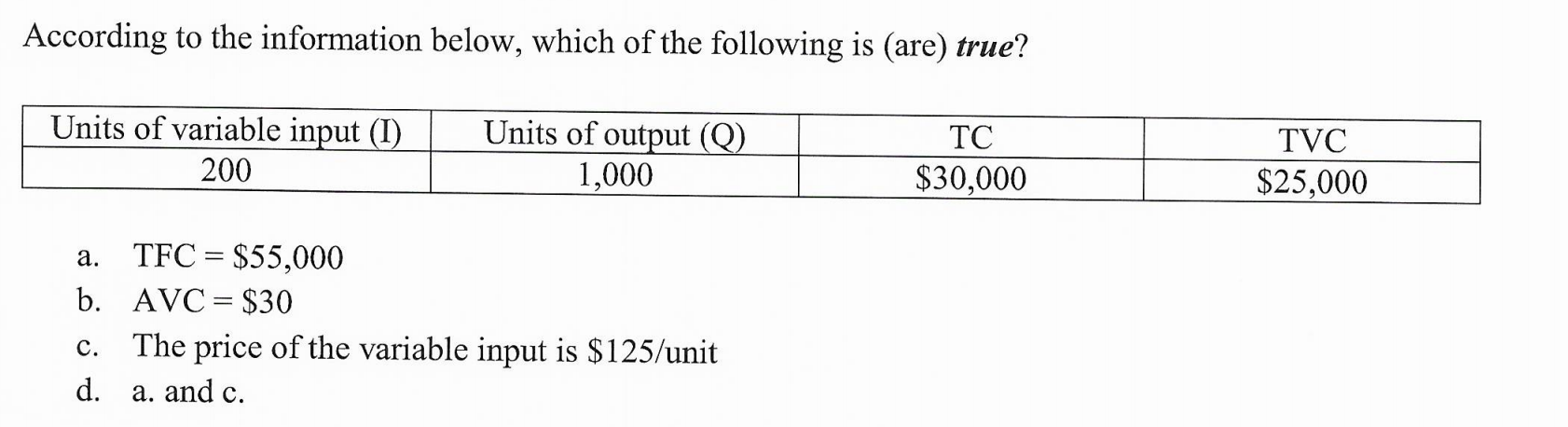# According to the information below, which of the following is (are) true?Units of variable input (I)Units of output (Q)1,000ТCTVC200\$30,000\$25,000TFC \$55,000b. AVC \$30The price of the variable input is \$125/unitа.с.d.a. and c

Question
1 viewshelp_outlineImage TranscriptioncloseAccording to the information below, which of the following is (are) true? Units of variable input (I) Units of output (Q) 1,000 ТC TVC 200 \$30,000 \$25,000 TFC \$55,000 b. AVC \$30 The price of the variable input is \$125/unit а. с. d. a. and c fullscreen
check_circle

Step 1

The total cost of production is the summation of all the fixed cost as well as the variable costs in the process of production. The fixed costs are the costs which remains the same at all the levels of output. In this case, the fixed cost will be the same when the output is zero or 1,000. The variable cost is the cost which varies as the output increases. This is because of the variations in the level of input used to produce the output as output increases.

Step 2

The total cost is given to be \$30,000 which means the summation of the fixed costs as well as the variable costs to produce 1,000 units is \$30,000. It is also given that the total variable cost is equal to \$25,000 for producing 1,000 units which means that the price of the variable input can be calculated by dividing the total variable cost with the units of variable input used which is 200. Thus, it can be calculated as follows:

Step 3

The average variable cost can be calculated by dividing the ...

### Want to see the full answer?

See Solution

#### Want to see this answer and more?

Solutions are written by subject experts who are available 24/7. Questions are typically answered within 1 hour.*

See Solution
*Response times may vary by subject and question.
Tagged in